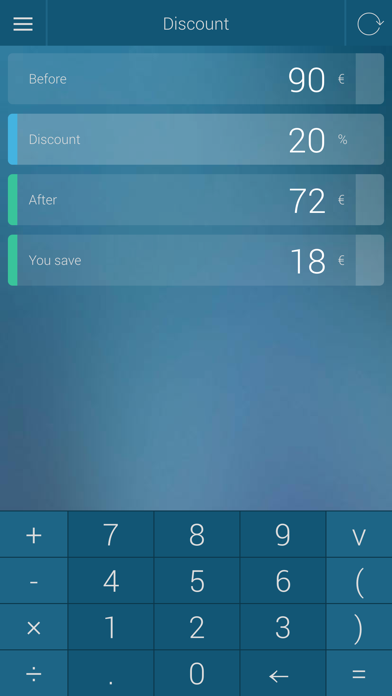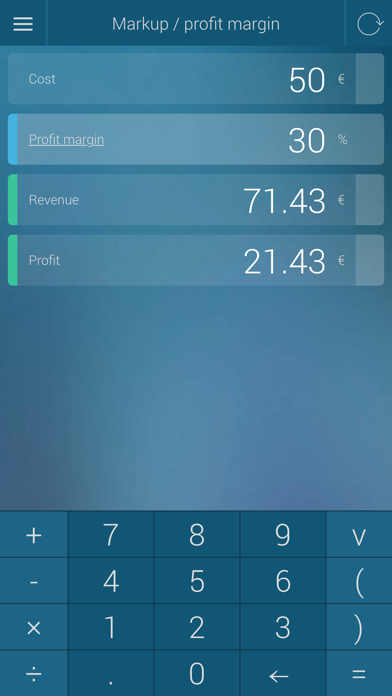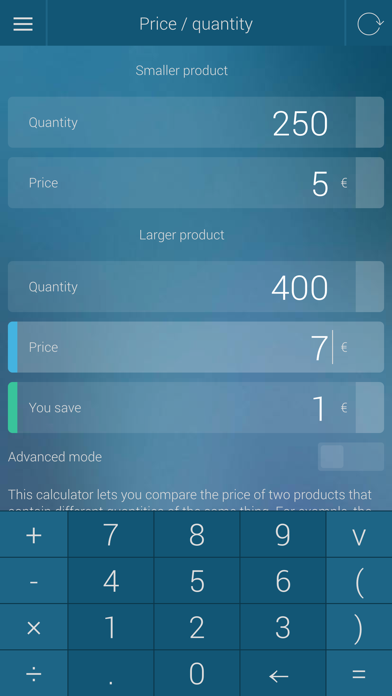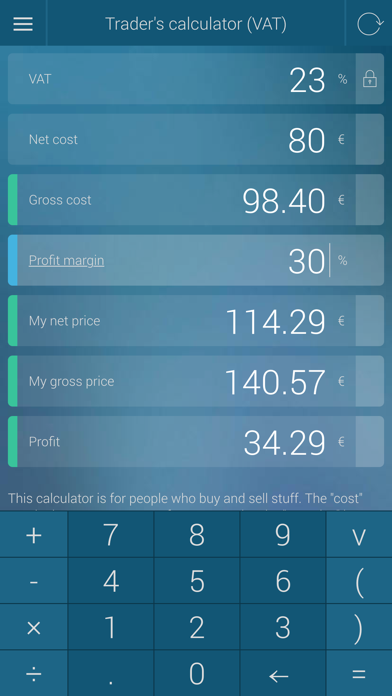## Percentage Calculator - percent, discount, tip - Lumos Educational App Store4.099991
Price -Free
\$0

#### DESCRIPTION:

Percentage Calculator is simply the best and easiest to use app which calculates: Everyday calculations simple percentage calculator (5 percent of 40 is 2) percentage increase/decrease (5 percent decrease from 40 is 38) tip calculator discount calculator convert fractions to percentages (5 / 20 is the same as 25 percent) Business calculators markup profit margin VAT Sales tax a powertul trader's calculator (VAT or sales tax, net cost, gross cost, markup/profit margin, my net price, my gross price and profit all in one calculator) compound interest

#### OVERVIEW:

Percentage Calculator - percent, discount, tip is a free educational mobile app By Omni Calculator Sp. z o.o..It helps students practice the following standards .

This page not only allows students and teachers download Percentage Calculator - percent, discount, tip but also find engaging Sample Questions, Videos, Pins, Worksheets, Books related to the following topics.

Developer: Omni Calculator Sp. z o.o.

Developer URL: NA

Software Version: 3.1.8

Category: Finance

Release Date: 2014-06-15T03:54:55Z#### Are you the Developer?EdSearch WebSearch